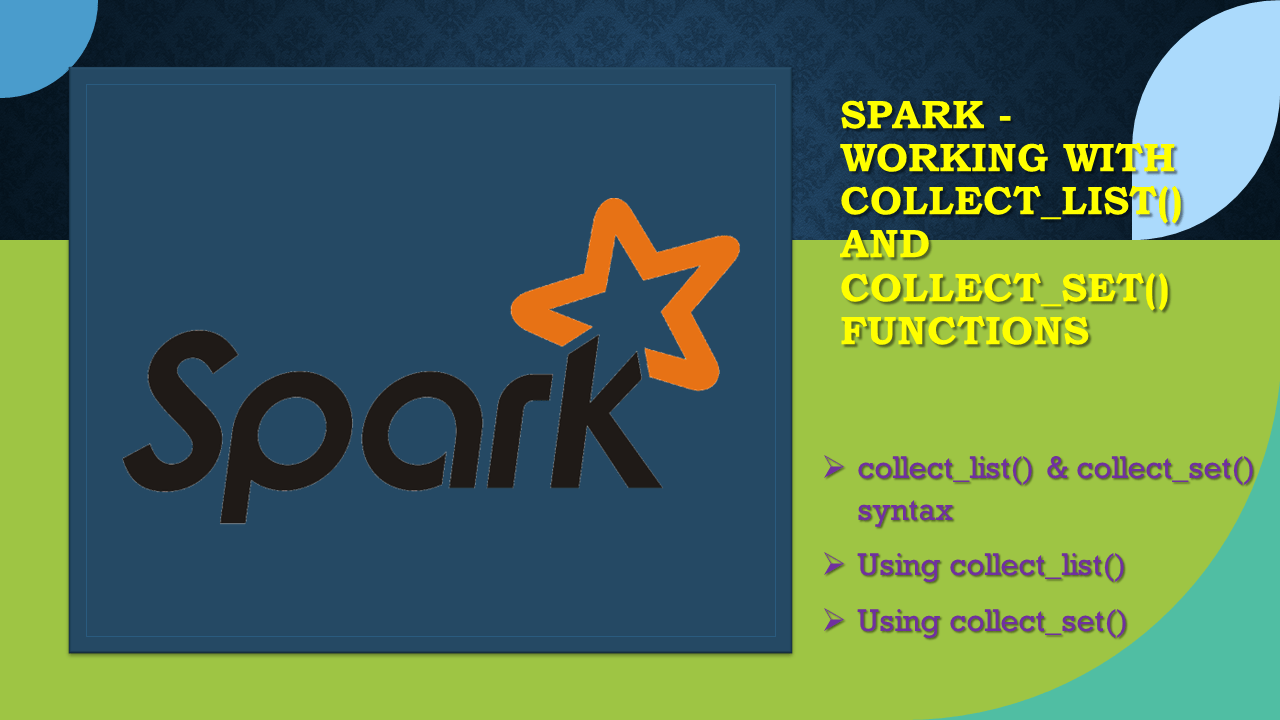# Spark – Working with collect_list() and collect_set() functions

Spark SQL `collect_list()` and `collect_set()` functions are used to create an array (ArrayType) column on DataFrame by merging rows, typically after group by or window partitions. In this article, I will explain how to use these two functions and learn the differences with examples.

In order to explain these with examples, first let’s create a DataFrame.

``````
val arrayStructData = Seq(
Row("James", "Java"), Row("James", "C#"),Row("James", "Python"),
Row("Michael", "Java"),Row("Michael", "PHP"),Row("Michael", "PHP"),
Row("Robert", "Java"),Row("Robert", "Java"),Row("Robert", "Java"),
Row("Washington", null)
)
val arrayStructSchema = new StructType().add("name", StringType)

val df = spark.createDataFrame(
spark.sparkContext.parallelize(arrayStructData),arrayStructSchema)
df.printSchema()
df.show(false)
``````

This yields below output

``````
// Output:
+----------+--------------+
|name      |booksInterested|
+----------+--------------+
|James     |Java          |
|James     |C#            |
|James     |Python        |
|Michael   |Java          |
|Michael   |PHP           |
|Michael   |PHP           |
|Robert    |Java          |
|Robert    |Java          |
|Robert    |Java          |
|Washington|null          |
+----------+--------------+
``````

## 1. collect_list() & collect_set() syntax

Spark colelct_list() and collect_set() is as follow

``````
def collect_list(e : org.apache.spark.sql.Column) : org.apache.spark.sql.Column
def collect_list(columnName : scala.Predef.String) : org.apache.spark.sql.Column
def collect_set(e : org.apache.spark.sql.Column) : org.apache.spark.sql.Column
def collect_set(columnName : scala.Predef.String) : org.apache.spark.sql.Column
``````

## 2. Using collect_list()

The Spark function `collect_list()` is used to aggregate the values into an ArrayType typically after group by and window partition.

In our example, we have a column name and booksInterested, if you see the James like 3 books and Michael likes 2 books (1 book duplicate) Now, let’s say you wanted to group by name and collect all values of booksInterested as an array. This is achieved first by grouping on “name” and aggregating on booksInterested.

Note that `colelct_list()` collects and includes all duplicates.

``````
// Using collect_list()
val df2 = df.groupBy("name").agg(collect_list("booksIntersted")
.as("booksInterested"))
df2.printSchema()
df2.show(false)
``````

This yields below output

``````
// Output:
root
|-- name: string (nullable = true)
|-- booksInterested: array (nullable = true)
|    |-- element: string (containsNull = true)

+----------+------------------+
|name      |booksInterested   |
+----------+------------------+
|James     |[Java, C#, Python]|
|Washington|[]                |
|Michael   |[Java, PHP, PHP]  |
|Robert    |[Java, Java, Java]|
+----------+------------------+
``````

## 3. Using collect_set()

Spark SQL function `collect_set()` is similar to `collect_list()` with difference being, collect_set() dedupe or eliminates the duplicates and results in unique for each value.

``````
// Using collect_set()
df.groupBy("name").agg(collect_set("booksInterested")
.as("booksInterestd"))
.show(false)
``````

This yields below utput

``````
// Output:
+----------+------------------+
|name      |booksInterested    |
+----------+------------------+
|James     |[Java, C#, Python]|
|Washington|[]                |
|Michael   |[PHP, Java]       |
|Robert    |[Java]            |
+----------+------------------+
``````

## 4. Complete example

``````
import org.apache.spark.sql.functions._
import org.apache.spark.sql.types.{StringType, StructType}
import org.apache.spark.sql.{Row, SparkSession}

object CollectListExample extends App {

val spark = SparkSession.builder().appName("SparkByExamples.com")
.master("local")
.getOrCreate()

val arrayStructData = Seq(
Row("James", "Java"), Row("James", "C#"),Row("James", "Python"),
Row("Michael", "Java"),Row("Michael", "PHP"),Row("Michael", "PHP"),
Row("Robert", "Java"),Row("Robert", "Java"),Row("Robert", "Java"),
Row("Washington", null)
)
val arrayStructSchema = new StructType().add("name", StringType)

val df = spark.createDataFrame(
spark.sparkContext.parallelize(arrayStructData),arrayStructSchema)
df.printSchema()
df.show(false)

val df2 = df.groupBy("name").agg(collect_list("booksIntersted")
.as("booksIntersted"))
df2.printSchema()
df2.show(false)

df.groupBy("name").agg(collect_set("booksIntersted")
.as("booksIntersted"))
.show(false)
}
``````

## Conclusion

In summary, Spark SQL function `collect_list()` and `collect_set()` aggregates the data into a list and returns an ArrayType. collect_set() de-dupes the data and return unique values whereas collect_list() return the values as is without eliminating the duplicates.

### Naveen (NNK)

Naveen (NNK) is a Data Engineer with 20+ years of experience in transforming data into actionable insights. Over the years, He has honed his expertise in designing, implementing, and maintaining data pipelines with frameworks like Apache Spark, PySpark, Pandas, R, Hive and Machine Learning. Naveen journey in the field of data engineering has been a continuous learning, innovation, and a strong commitment to data integrity. In this blog, he shares his experiences with the data as he come across. Follow Naveen @ @ LinkedIn

### This Post Has 4 Comments

1.Rajesh

Note that colelct_list() preserves the order it collects. – sparkbyexamples

As mentioned in the above post, is colelct_list() really preserves the order?

In my production scenario, I found that colelct_list() is not preserving the order. The value from the 2nd row is adding to the array as the “first value” and the value from the 1st row is adding to the array as the “second value”.

1.NNK

Hi Rajesh, I’ve tried this myself and agree with you collect_list() doesn’t preserve the order. Thanks for your comment.

1.susant

Hello, collect_set() is taking long time as it’s involved grouping/aggregation. is there an other way to get the same result as collect_set(). Thanks in advance

2.Anonymous

Can we do a collect_set() for arrary type data in the columns.